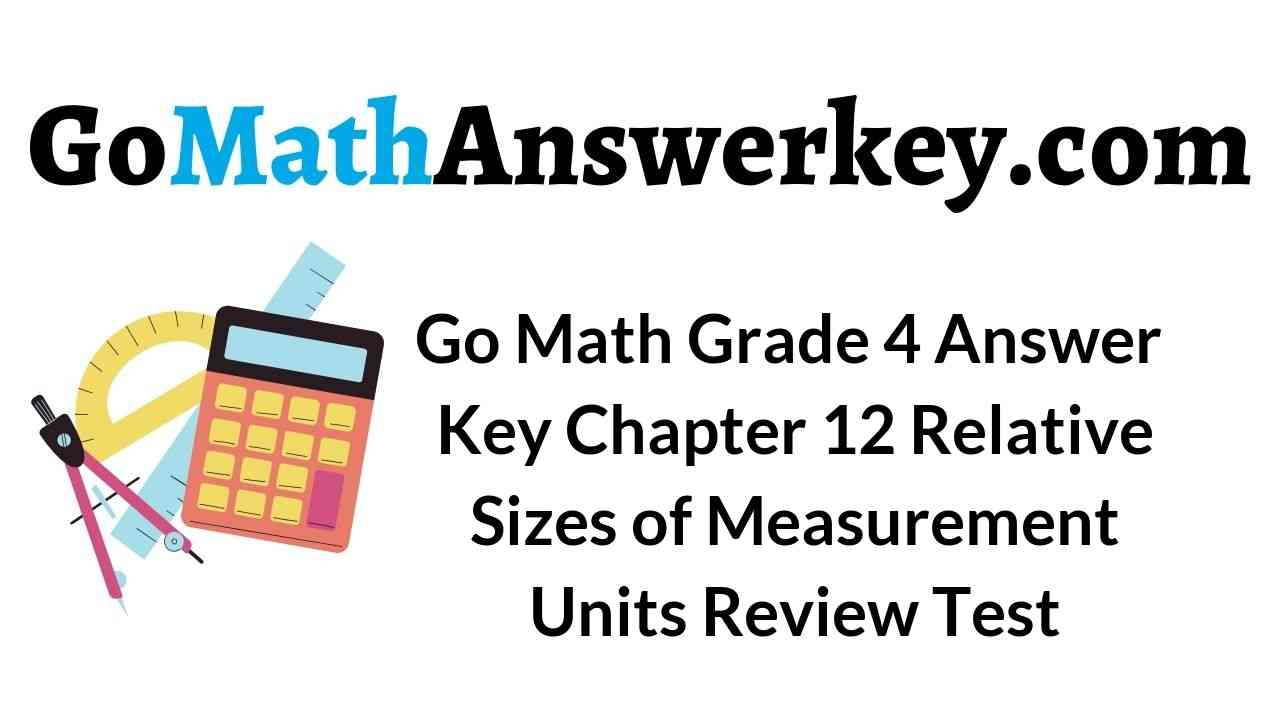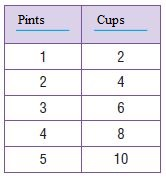# Go Math Grade 4 Answer Key Homework FL Chapter 12 Relative Sizes of Measurement Units Review/Test

Enhance your math skills by referring to the Go Math Grade 4 Answer Key Homework FL Chapter 12 Relative Sizes of Measurement Units Review/Test. With the help of this HMH Go Math Grade 4 Review/Test Answer Key you score good marks in the exam.

## Go Math Grade 4 Answer Key Homework FL Chapter 12 Relative Sizes of Measurement Units Review/Test

Go Math Grade 4 Answer Key Homework FL Review/Test covers all the topics in Chapter 12 Relative Sizes of Measurement Units. Test the knowledge of your child by giving the question from Review/Test. Just click on the link and Download Go Math Grade 4 Solution Key Homework FL Chapter 12 Relative Sizes of Measurement Units Review/Test pdf.

Chapter 12 – Review/Test

### Review/Test – Page No. 491

Choose the best term from the box to complete the sentence.Question 1.
A ___________ is a metric unit for measuring length or distance.
________

A millimeter is a metric unit for measuring length or distance.

Question 2.
A ___________ is a metric unit for measuring liquid volume.
________

A milliliter is a metric unit for measuring liquid volume.

Question 3.
A ___________ is a graph that shows the frequency of data along a number line.
________

A line plot is a graph that shows the frequency of data along a number line.

Question 4.
A ___________ is a customary unit for measuring liquid volume.
________

A quart is a customary unit for measuring liquid volume.

Complete.

Question 5.
9 feet = _____ inches

Explanation:
Convert from feet to inches
1 feet = 12 inches
9 feet = 9 × 12 inches = 108 inches
Thus 9 feet = 108 inches

Question 6.
7 tons = _____ pounds

Explanation:
Converting from tons to pounds
1 ton = 2000 pounds
7 tons = 7 × 2000 pounds = 14,000 pounds
Thus 7 tons = 14,000 pounds

Question 7.
10 pints = _____ cups

Explanation:
Converting from pints to cups.
1 pint = 2 cups
10 pints = 10 × 2 cups = 20 cups
Thus 10 pints = 20 cups

Question 8.
4 decimeters = _____ centimeters

Explanation:
Converting from decimeters to centimeters.
1 decimeter = 10 centimeter
4 decimeters = 4 × 10 centimeter = 40 centimeters
Thus 4 decimeters = 40 centimeters

Question 9.
8 liters = _____ milliliters

Explanation:
Converting from liters to milliliters.
1 liter = 1000 milliliters
8 liters = 8 × 1000 milliliters
= 8000 milliliters
Thus 8 liters = 8000 milliliters

Question 10.
5 weeks = _____ days

Explanation:
Converting from weeks to days.
1 week = 7 days
5 weeks = 5 × 7 days = 35 days
Thus 5 weeks = 35 days

Compare using <, >, or =.

Question 11.
3 yards _____ 36 inches

Explanation:
Converting from yards to inches.
1 yard = 36 inches
3 yards = 108 inches
Thus 3 yards > 36 inches

Question 12.
10 cups _____ 80 fluid ounces

Explanation:
Converting from cups to fluid ounces.
1 cup = 8 fluid ounces
10 cups = 8 × 10 = 80 fluid oiunces
Thus 10 cups = 80 fluid ounces

Question 13.
4 pounds _____ 96 ounces

Explanation:
Converting from pounds to ounces.
1 pound = 16 ounces
4 pounds = 4 × 16 ounces = 64 ounces
64 ounces is less than 96 ounces
Thus, 4 pounds < 96 ounces

Question 14.
8 meters _____ 700 centimeters

Explanation:
Converting from meters to centimeters.
1 meter = 100 centimeters
8 meters = 8 × 100 centimeters = 800 centimeters
800 centimeters is greater than 700 centimeters.
Thus, 8 meters > 700 centimeters

Question 15.
6 liters _____ 6,500 milliliters

Explanation:
Converting from liters to milliliters.
1 liter = 1000 milliliters
6 liters = 6 × 1000 milliliters
6000 milliliters is less than 6500 milliliters.
Thus, 6 liters < 6,500 milliliters.

Question 16.
9 kilograms _____ 9,000 grams

Explanation:
Converting from kilograms to grams.
1 kilogram = 1000 grams
9 kilograms = 9 × 1000 grams = 9000 grams
9 kilograms = 9,000 grams

Question 17.
8 hr 30 min
− 6 hr 25 min
————————–
_____ hr _____ min

Explanation:
8 hr 30 min
-6 hr 25 min
2 hr 5 min

Question 18.
7 c 4 fl oz
+4 c 3 fl oz
———————–
_____ c _____ fl oz

Explanation:
7 c 4 fl oz
+4 c 3 fl oz
11 c 7 fl oz.

Question 19.
9 yd 1 ft
−5 yd 2 ft
———————–
_____ yd _____ ft

Explanation:
First, convert from the yard to feet.
1 yard = 3 ft
9 yd 1 ft = 8 yd 4 ft
8 yd 4 ft
-5 yd 2 ft
3 yd 2 ft

### Review/Test – Page No. 492

Question 20.
Maya’s band rehearsal started at 10:30 A.M. It ended 1 hour and 40 minutes later. At what time did Maya’s band rehearsal end?
Options:
a. 12:10 A.M.
b. 8:50 A.M.
c. 12:10 P.M.
d. 11:10 P.M.

Explanation:
Given,
Maya’s band rehearsal started at 10:30 A.M. It ended 1 hour and 40 minutes later.
10 hr 30 min
+1 hr 40 min
11 hr 70 min
Now convert 70 min to hours.
70 min = 1 hr 10 min
11 hr 70 min = 12:10 P.M.
Thus the correct answer is option C.

Question 21.
Darlene is making punch. She pours 4 quarts 2 cups of apple juice into a bowl. Then she pours 3 quarts 1 cup of grape juice into the bowl. How much juice is in the bowl now?
Options:
a. 1 quart 1 cup
b. 7 quarts 1 cup
c. 7 quarts 3 cups
d. 8 quarts 1 cup

Explanation:
Given,
Darlene is making punch. She pours 4 quarts 2 cups of apple juice into a bowl.
Then she pours 3 quarts 1 cup of grape juice into the bowl.
4 quarts 2 cups
+3 quarts 1 cup
7 quarts 3 cups
Thus the correct answer is option c.

Question 22.
Kainoa bought a brick of modeling clay that was labeled 2 kilograms. He needs to separate the clay into balls that are measured in grams. How many grams does he have?
Options:
a. 20 grams
b. 200 grams
c. 2,000 grams
d. 20,000 grams

Explanation:
Given,
Kainoa bought a brick of modeling clay that was labeled 2 kilograms.
He needs to separate the clay into balls that are measured in grams.
Convert from kilograms to grams.
1 kilogram = 1000 grams
2 kilograms = 2 × 1000 grams = 2000 grams
Thus the correct answer is option c.

Question 23.
A truck driver’s truck weighs 3 tons. A weigh station measures the weight in pounds. How many pounds does the truck weigh?
Options:
a. 600 pounds
b. 2,000 pounds
c. 3,000 pounds
d. 6,000 pounds

Explanation:
Given,
A truck driver’s truck weighs 3 tons. A weigh station measures the weight in pounds.
Convert from tons to pounds.
1 ton = 2000 pounds
3 tons = 3 × 2000 pounds = 6000 pounds
Thus the correct answer is option d.

### Review/Test – Page No. 493

Question 24.
Brody and Amanda canoed for 1 hour and 20 minutes before stopping to fish at 1:15 P.M. At what time did they start canoeing?
Options:
a. 11:55 A.M.
b. 12:05 P.M.
c. 2:35 P.M.
d. 11:55 P.M.

Explanation:
Given,
Brody and Amanda canoed for 1 hour and 20 minutes before stopping to fish at 1:15 P.M.
13 hr 15 min
-1 hr 20 min
11 hr 55 min
Thus they start canoeing at 11:55 A.M.
Thus the correct answer is option d.

Question 25.
Lewis fills his thermos with 2 liters of water. Garret fills his thermos with 1 liter of water. How many more milliliters of water does Lewis have than Garret?
Options:
a. 1 more milliliter
b. 100 more milliliters
c. 1,000 more milliliters
d. 2,000 more milliliters

Explanation:
Given,
Lewis fills his thermos with 2 liters of water. Garret fills his thermos with 1 liter of water.
2 liters
-1 liters
1 liter
Convert from liters to milliliters.
1 liter = 1000 milliliters
Thus the correct answer is option c.

Question 26.
Lola won the 100-meter freestyle event at her swim meet. How many decimeters did Lola swim?
Options:
a. 1 decimeter
b. 10 decimeter
c. 100 decimeter
d. 1,000 decimeter

Explanation:
Given,
Lola won the 100-meter freestyle event at her swim meet.
Convert from meter to decimeter.
1 meter = 10 decimeter
100 meter = 100 × 10 decimeter = 1000 decimeter
Thus the correct answer is option d.

Question 27.
What is the best estimate for the length of an ant’s leg?
Options:
a. 2 millimeters
b. 2 centimeters
c. 2 decimeters
d. 2 meters

Explanation:
The best estimation for the length of an ant’s leg is 2 centimeters.
Thus the correct answer is option b.

### Review/Test – Page No. 494

Question 28.
Sabita made this table to relate two customary units of liquid volume. List the number pairs for the table. Describe the relationship between the numbers in each pair.Type below:
________

Answer: The relationship between the numbers in each pair is pints and cups.

Question 29.
Type below:
________Question 30.
Landon borrowed a book from the library. The data show the lengths of time Landon read the book each day until he finished it.A. Make a tally table and a line plot to show the data.Type below:
________Question 30.
B. Explain how you used the tally table to label the numbers and plot the Xs on the line plot.
Type below:
________

I used the number of tallys to the plot the Xs on the line plot.

Question 30.
C. What is the difference between the longest time and shortest time Landon spent reading the book?
$$\frac{□}{□}$$ hour

Answer: $$\frac{3}{4}$$ hour

Explanation:
The shortest time Landon spent reading the book is 1/4
The longest time Landon spent reading the book is 1
1 – 1/4 = 3/4
Thus the difference between the longest time and shortest time Landon spent reading the book is $$\frac{3}{4}$$ hour.

Conclusion:

The students of 4th grade can avail all chapters Go Math Grade Answer Key in pdf format so that your learning will kick start in an effective manner. We have given a brief explanation of each and every question on our Go Math Grade 4 Answer Key Chapter 12 Relative Sizes of Measurement Units. We suggest the students understand the concepts and apply them in the real world.

Scroll to Top